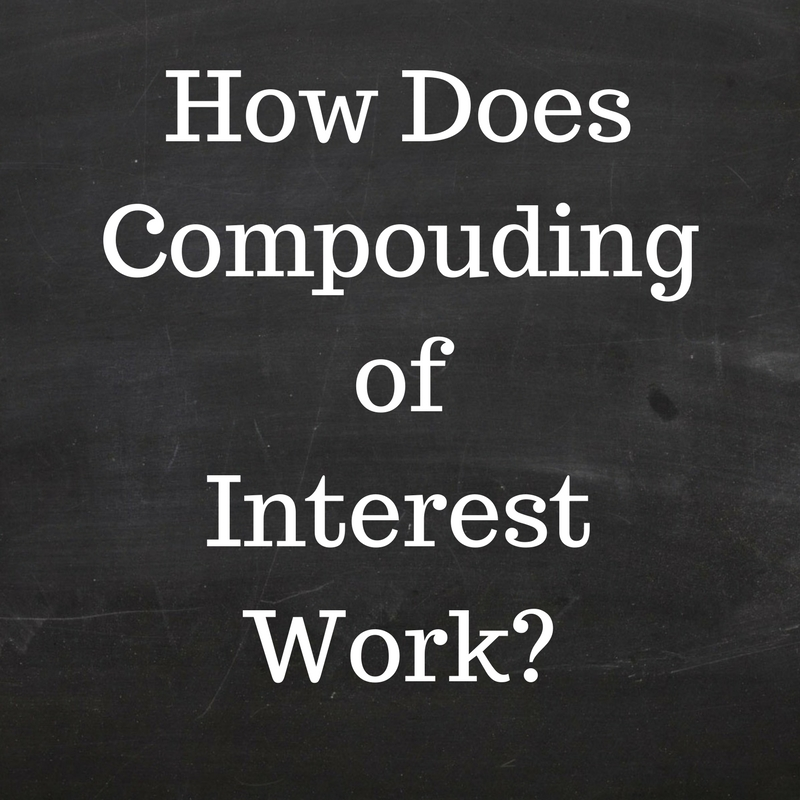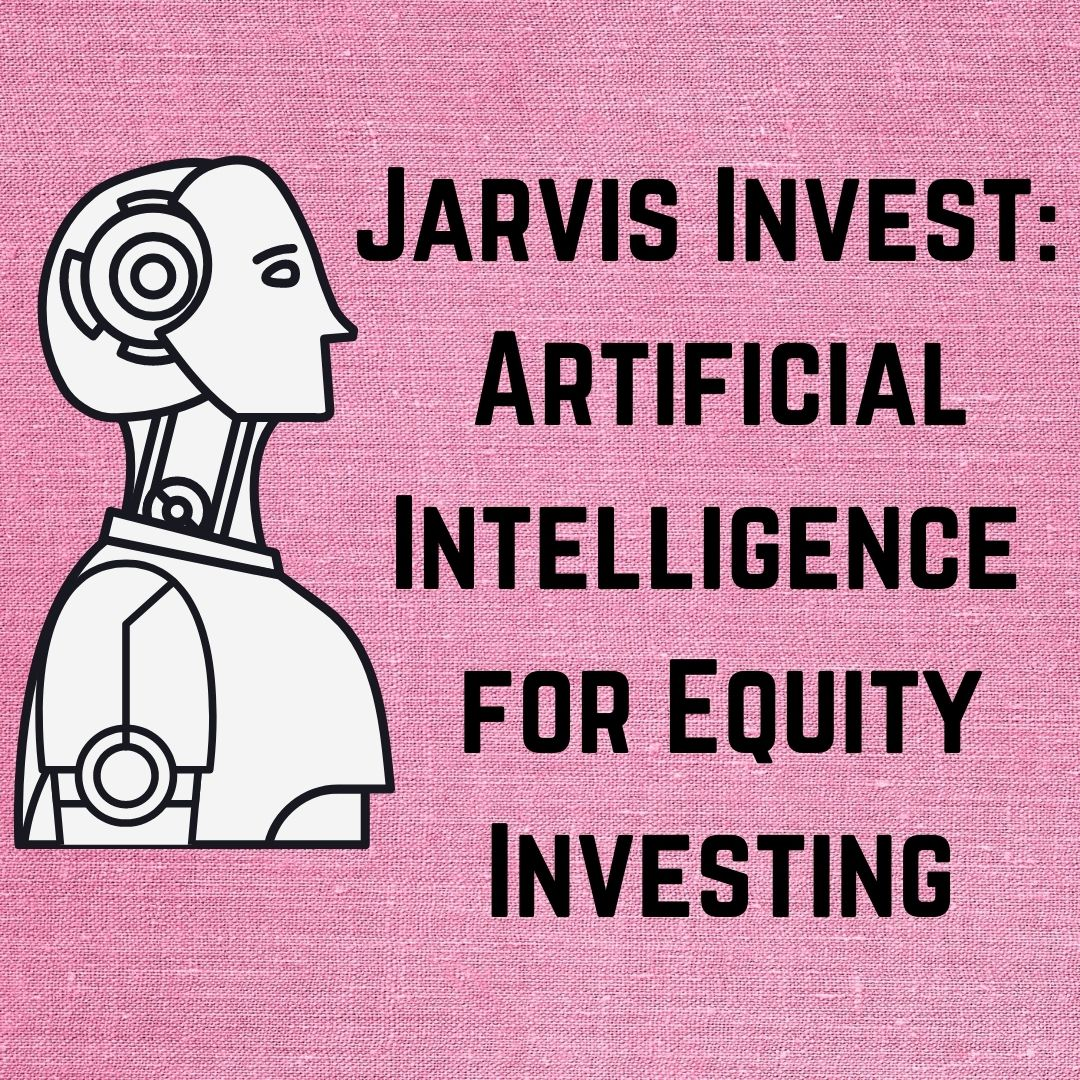# How Does Compounding of Interest Work?Compounding is the process and the ability of an asset to generate earnings from the previous earnings. Compounding is where the investment value rises because the earnings on an investment, both capital gains and interest, earn interest as time passes.  Compounding (sometimes called as “compound interest”) transform you are working money into a highly powerful income-generating tool. To work, it requires two concepts: the time and the reinvestment of earnings. The more time you will give to your investments, the more you are able to gain the income potential of your original investment.

Compound interest is interest paid on the accumulated interest on money you have borrowed or invested as well as the interest paid on the initial principal. Compound interest is just like double chocolate topping for your savings. You will earn interest on the money that you have deposited, and on the interest that you have already earned though you earn interest on interest. An online savings account paying you the monthly interest is a very good example of an account that earns compound interest on interest.

Here is an example of compound interest, suppose you deposit \$4000 in the bank. If interest is paid is 5% annually, then the bank will give you about \$200 in interest for the first year of your investment. If you leave that 200in your bank account, it will start earning interest as well. The following year, your \$4200 principal balance will earn \$210 (4200*5/100) in interest. Over time, this observable fact turns into the great magic of compound interest.

Investors should also note that the rate of compounding may be decreased or increased, depending on how the interest amount is paid and calculated. The quicker the interval between interest calculations, the lower interest will accrue and the shorter the interval between interest calculations, the faster interest will accrue. Therefore, the portion which calculates and pays interest on a daily basis will grow more rapidly than the same account calculating interest on a monthly basis.

There are two types of interest: Simple interest is interest paid on the principal capital only and Compound interest that is paid on the principal, plus the accumulating interest.

The Compound interest is calculated below by the following formula:.

Use the formula A = P x (1 + r/n)^nt

 ‘A’ = end amount of your investment ‘P’ = principal, i.e. the starting amount ‘r’ = percentage interest rate converted to a decimal rate ‘n’ = number of time periods

T  = the time involved, in years.

The longer money compounds, the quicker it grows. Money growing at 6 % per year will double in about 12 years, but it will be value you 4 times as much in 24 years. Compound Interest could be calculated per month, per day, or per year.

Thus, compounding is a powerful force. How do compounding workout and how it might work for you? Fortunately, there is a very easiest rule of thumb to help you calculate compound interest. Rule of thumb called the Rule of 72. This is how it works.

Like take the interest rate you expect to earn and divide it into 72 and the answer is the number of years it will take to double your money. So at 6% a year it will take 12 years for you to double your money. A 3% return will take 24 years to get doubled and at 12% it will only take 6 years. Compounding also works in the reverse direction. If you are applying compound interest for a loan rather than applying an interest rate a deposit without either servicing the interest or paying down the capital the amount outstanding will grow and grow. Suppose, if you take out a loan of £200 (or any other currency) with an interest rate of 10 per cent a year and don’t repay anything, you would owe £220 after one year, £242 after two years and £ 266.2 after three years.

It really doesn’t matter if you are putting some money into low rate savings accounts or short term or CDs or long-term, higher return investments but still compound interest will work for your benefit if you let it.

Compound interest is best when it will work in your favor in investments, but it can also be your biggest enemy when it works against you or put you in loss in loans and other debts. It’s up to an individual how you can let it work in favor.

Compound interest can be your best friend if you stay on top of your loan payments and always keep an eye on your investments, then it comes to wealth.

## Related Posts